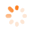# node selection with keypress

TreeViewTop achievementsRank 1
Tom M asked on 20 Oct 2010, 09:34 PM
Greetings All,

I am looking for a way to select a node using a keystoke. In other words, if the user presses the "R" key while focused on the RadTree the first node with text that starts with "R" would be selected. I have a requirement that needs this quick navigation. I was looking at the OnClientKeyPressing event and think I'll be able to use this, but was just wondering if anyone has done this before and has a good approach.

Regards,
Tom

## 2 Answers, 1 is accepted

0Shinu
Top achievementsRank 2
answered on 21 Oct 2010, 08:33 AM
Hello Tom,

RadTreeView supports keyboard navigation. To enable keyboard support of RadTreeView you only have to set the TabIndex or AccessKey property.

For selecting a particular node, on pressing the key, I guess the client side approach is better.

-Shinu.
0Tom M
Top achievementsRank 1
answered on 22 Oct 2010, 02:40 PM

Thanks for your response, Shinu. I guess I am going to take the client side approach. I've got something like this so far. It doesn't work great, because it only gets you to the first occurrence of a given character ( i.e. the first node with text that starts with "C" or whatever key you press) but its a start.

Regards,
Tom

`function` `OnGPKeyPressing(sender, args) { `
`        ``var` `character = ``null``; `
`        ``var` `node = args.get_node(); `
`        ``var` `nodes = gpTree.get_nodes(); `
`        ``var` `key = args.get_domEvent().keyCode; `
`        ``switch` `(key) { `
`            ``case` `65: character = ``"A"``; ``break``; `
`            ``case` `66: character = ``"B"``; ``break``; `
`            ``case` `67: character = ``"C"``; ``break``; `
`            ``case` `68: character = ``"D"``; ``break``; `
`            ``case` `69: character = ``"E"``; ``break``; `
`            ``case` `70: character = ``"F"``; ``break``; `
`            ``case` `71: character = ``"G"``; ``break``; `
`            ``case` `72: character = ``"H"``; ``break``; `
`            ``case` `73: character = ``"I"``; ``break``; `
`            ``case` `74: character = ``"J"``; ``break``; `
`            ``case` `75: character = ``"K"``; ``break``; `
`            ``case` `76: character = ``"L"``; ``break``; `
`            ``case` `77: character = ``"M"``; ``break``; `
`            ``case` `78: character = ``"N"``; ``break``; `
`            ``case` `79: character = ``"O"``; ``break``; `
`            ``case` `80: character = ``"P"``; ``break``; `
`            ``case` `81: character = ``"Q"``; ``break``; `
`            ``case` `82: character = ``"R"``; ``break``; `
`            ``case` `83: character = ``"S"``; ``break``; `
`            ``case` `84: character = ``"T"``; ``break``; `
`            ``case` `85: character = ``"U"``; ``break``; `
`            ``case` `86: character = ``"V"``; ``break``; `
`            ``case` `87: character = ``"W"``; ``break``; `
`            ``case` `88: character = ``"X"``; ``break``; `
`            ``case` `89: character = ``"Y"``; ``break``; `
`            ``case` `90: character = ``"Z"``; ``break``; `
`            ``default``: character = ``"other"``; ``return``; `
`        ``} `
`        ``for` `(``var` `i = 0; i < gpTree.get_nodes().get_count(); i++) { `
`            ``var` `node = gpTree.get_nodes().getNode(i); `
`            ``if` `(node.get_text().charAt(0) == character && !node.get_selected()) { `
`                ``gpTree.trackChanges(); `
`                ``gpTree.get_selectedNode().toggle(); `
`                ``node.get_element().scrollIntoView(); `
`                ``gpTree.commitChanges(); `
`                ``node.select(); `
`                ``break``; `
`            ``} `
`        ``} `
`    ``}`
Tags
TreeViewTom M
Top achievementsRank 1Rank 2Rank 1